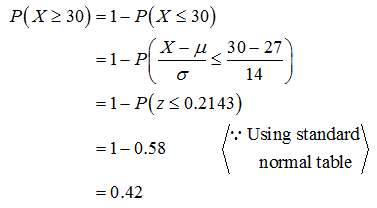# Los Angeles workers have an average commute of 27 minutes. Suppose the LA commute time is normally distributed with a standard deviation of 14 minutes. Let X represent the commute time for a randomly selected LA worker. Round all answers to 4 decimal places where possible.a. What is the distribution of X? X ~ N(,) b. Find the probability that a randomly selected LA worker has a commute that is longer than 30 minutes. c. Find the 85th percentile for the commute time of LA workers. minutes

Question
8 views

Los Angeles workers have an average commute of 27 minutes. Suppose the LA commute time is normally distributed with a standard deviation of 14 minutes. Let X represent the commute time for a randomly selected LA worker. Round all answers to 4 decimal places where possible.

a. What is the distribution of X? X ~ N(,)

b. Find the probability that a randomly selected LA worker has a commute that is longer than 30 minutes.

c. Find the 85th percentile for the commute time of LA workers. minutes

check_circle

Step 1

Let X be the commute time.

It is given that X is normally distributed with mean 27 minutes and standard deviation is 14 minutes.

a).

The distribution of X is given as X~N(µ=27, σ2=14).

Step 2

b).

The probability that a randomly selected LA worker has a commute that greater than 30 minutes is calculated as follows:...

### Want to see the full answer?

See Solution

#### Want to see this answer and more?

Solutions are written by subject experts who are available 24/7. Questions are typically answered within 1 hour.*

See Solution
*Response times may vary by subject and question.
Tagged in